# Mensuration 3D

• Difficulty Level : Medium
• Last Updated : 04 Feb, 2022

Mensuration 3D deals with shapes like cube, cuboid, sphere etc. The problems are generally based on volume and surface area.

### Cuboid

Let the length, breadth and height of the cuboid be ‘L’, ‘B’ and ‘H’ respectively.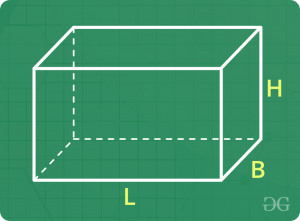• Volume = L x B x H
• Curved Surface area = 2 H (L + B)
• Total surface area = 2 (L B + B H + H L)
• Length of diagonal = (L2 + B2 + H2)1/2

### Cube

Let the side of the cube be ‘a’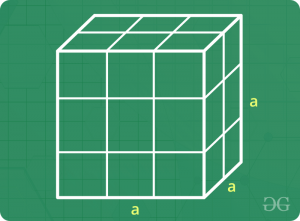• Volume = a3
• Curved Surface area = 4 a2
• Total surface area = 6 a2
• Length of diagonal =a

### Cylinder (Right Circular Cylinder)

Let the radius of the base and height of the right circular cylinder be ‘R’ and ‘H’ respectively.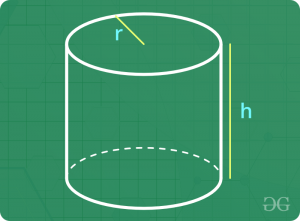• Volume = π R2 H
• Curved Surface area = 2 π R H
• Total surface area = 2 π R H + 2 π R2

### Hollow Cylinder (Hollow Right Circular Cylinder)

Let the inner radius of the base, outer radius of the base and height of the hollow right circular cylinder be ‘r’, ‘R’ and ‘H’ respectively.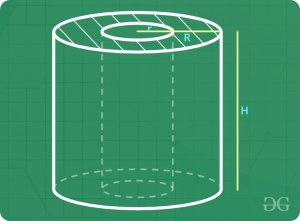• Volume = π H (R2 – r2)
• Curved Surface area = 2 π R H + 2 π r H = 2 π H (R + r)
• Total surface area = 2 π H (R + r) + 2 π (R2 – r2)

### Cone

Let the radius of the base, slant height and height of the cone be ‘R’, ‘L’ and ‘H’ respectively.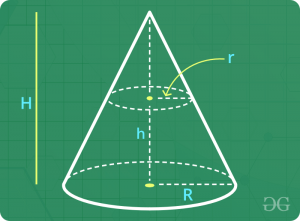• L2 = R2 + H2
• Volume = π R2 H / 3
• Curved Surface area = π R L
• Total surface area = π R L + π R2

### Sphere

Let the radius of the sphere be ‘R’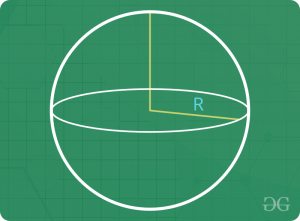• Volume = (4 / 3) π R3
• Surface area = 4 π R2

### Hemisphere

Let the radius of the hemisphere be ‘R’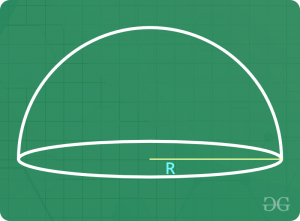• Volume = (2 / 3) π R3
• Curved Surface area = 2 π R2
• Total Surface area = 3 π R2

Please note that whenever it is mentioned to find “Surface Area”, we calculate the total surface area.

### Sample Problems

Question 1 : Find the length of the largest rod that can be kept in a cuboidal room of dimensions 10 x 15 x 6 m.
Solution : Largest rod would lie along the diagonal.
=> Length of largest rod = Length of diagonal of the room = (L2 + B2 + H2)1/2
=> Length of the largest rod = (102 + 152 + 62)1/2 = (100 + 225 + 36)1/2 = (361)1/2
=> Length of the largest rod = 19 m

Question 2 : Find the number of bricks of dimension 24 x 12 x 8 cm each that would be required to make a wall 24 m long, 8 m high and 60 cm thick.
Solution : Volume of 1 brick = 24 x 12 x 8 = 2304 cm 3
Volume of wall = 2400 x 800 x 60 = 115200000 cm 3
Therefore, number of bricks required = 115200000 / 2304 = 50000

Question 3 : A rectangular sheet of paper measuring 22 cm x 7 cm is rolled along the longer side to make a cylinder. Find the volume of the cylinder formed.
Solution : Let the radius of the cylinder be ‘R’.
The sheet is rolled along the longer side.
=> 2 π R = 22
=> R = 3.5 cm
Also, height = 7 cm
Therefore, volume of the cylinder = π R2 H = π (3.5)2 7 = 269.5 cm3

Question 4 : If each edge of a cube is increased by 10 %, what would be the percentage increase in volume ?
Solution : Let the original edge length be ‘a’
=> Original volume = a3
Now, new edge length = 1.1 a
=> New volume = (1.1 a)3 = 1.331 a3
=> Increase in volume = 1.331 a3 – 1 a3 = 0.331 a3
Therefore, percentage increase int eh volume = (0.331 a3 / a3) x 100 = 33.1 %

Question 5 : Three metal cubes of edge length 3 cm, 4 cm, 5 cm are melted to form a single cube. Find the edge length of such cube.
Solution : Volume of new cube = Volume of metal generated on melting the cubes = Sum of volumes of the three cubes
=> Volume of new cube = 3 3 + 4 3 + 5 3 = 216
=> Edge length of new cube = (216)1/3 = 6 cm

Question 6 : Find the length of a 1.25 m wide metal sheet required to make a conical machine of radius 7 m and height 24 m.
Solution : The sheet would be shaped into cone.
=> Area of sheet = Area of conical machine
=> 1.25 x Length = π x R x L
=> 1.25 x Length = π x R x (72 + 242)1/2
=> 1.25 x Length = π x 7 x 25
=> Length = 440 m
Thus, 440 m long metal sheet is required to make the conical machine.

Question 7 : From a cylindrical vessel having radius of the base 7 cm and height 6cm, water is poured into small hemispherical bowls each of radius 3.5 cm. Find the minimum number of bowls that would be required to empty the cylindrical vessel.
Solution : Volume of cylindrical vessel = π R2 H = π (72) 6 = 924 cm3
Volume of each bowl = (2 / 3) π R3 = (2 / 3) π 3.53 = 269.5 / 3
=> Number of bowls required = (924) / (269.5 / 3) = 10.28
But since number of bowls cannot be in fraction, we need atleast 11 such bowls to empty the cylindrical vessel.

### Problems on Mensuration 3D | Set-2

This article has been contributed by Nishant Arora

Please write comments if you have any doubts related to the topic discussed above, or if you are facing difficulty in any question or if you would like to discuss a question other than those mentioned above.

Please write comments if you find anything incorrect, or you want to share more information about the topic discussed above

My Personal Notes arrow_drop_up
Recommended Articles
Page :